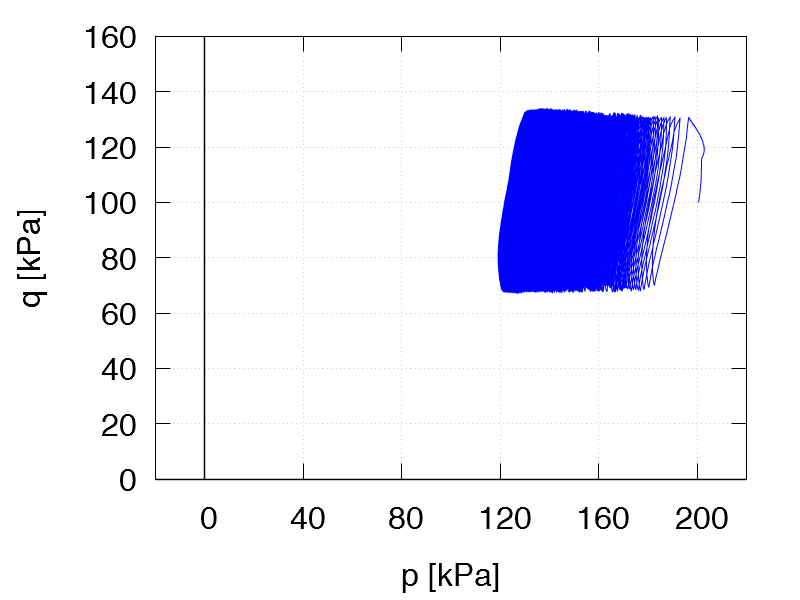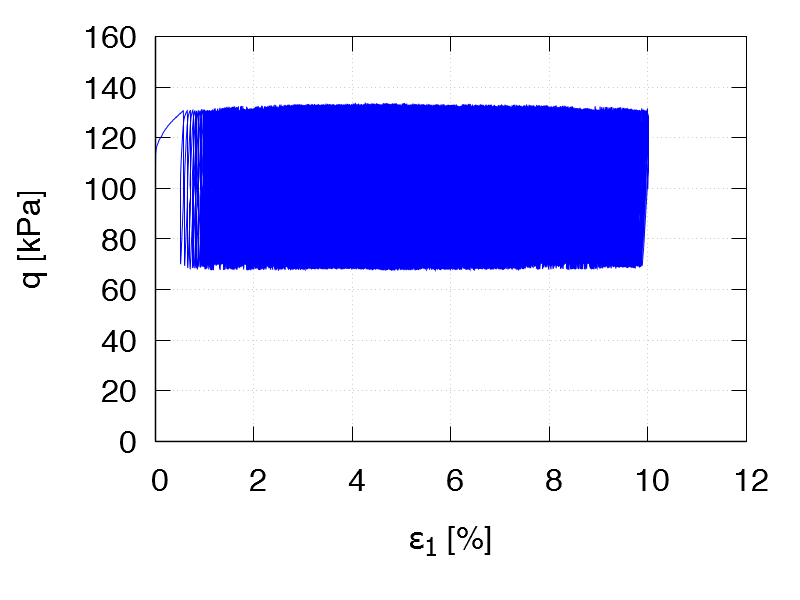Undrained cyclic tests with isotropic consolidation and stress cycles: Samples cut out in the horizontal direction   Data of all tests of this series

All tests: p0 = 200 kPa, Displacement rate 0.1 mm/min
Test C40
e0 = 1.125
η0 = 0
qampl = 45 kPa

Test data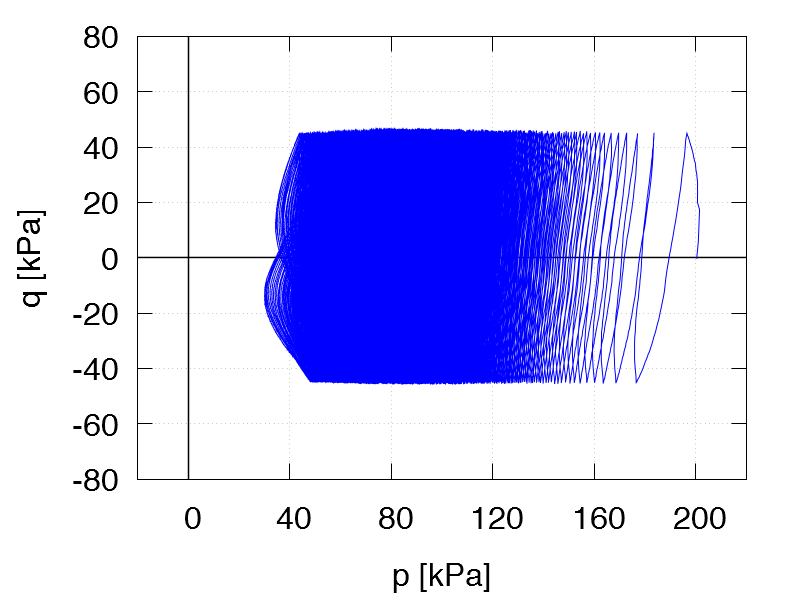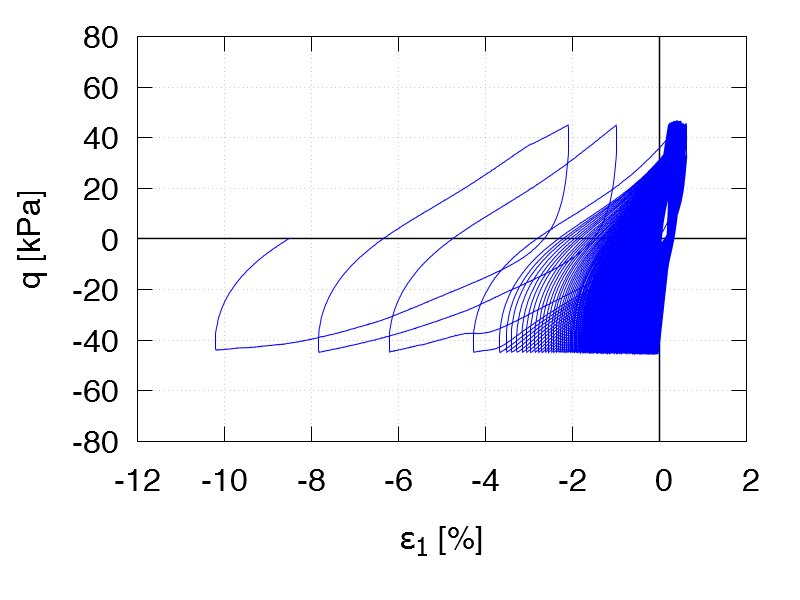Test C41
e0 = 1.252
η0 = 0
qampl = 45 kPa

Test data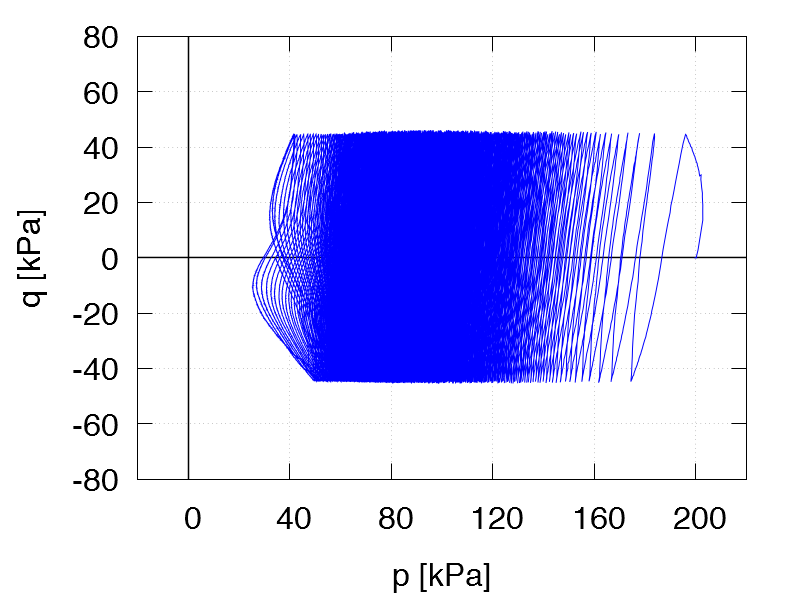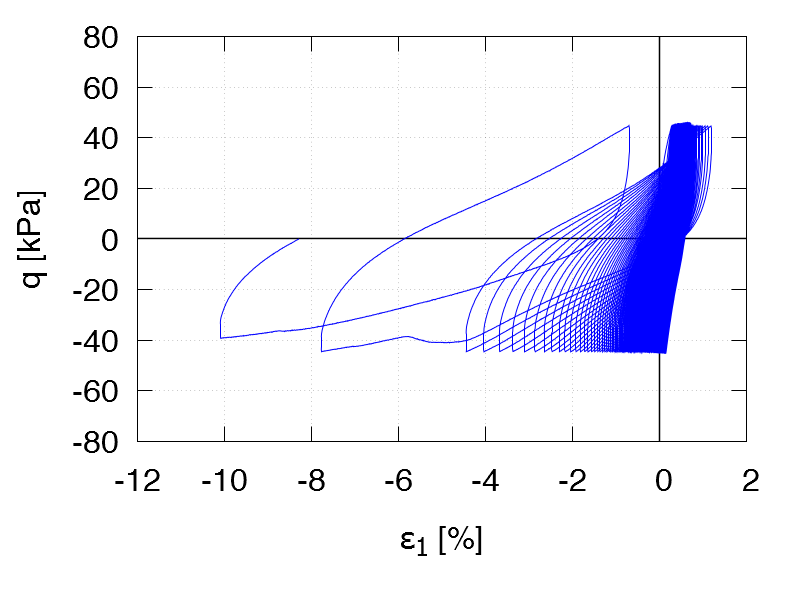Test C42
e0 = 1.100
η0 = 0.5
qampl = 30 kPa

Test data